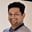Related Tags

general
communitycreator

# What is an adjacency list?Tarun Telang

## The need for an adjacency list

An adjacency matrix is a popular and simple way to represent a graph. However, it is most suitable when your model does not frequently manipulate vertices. Adjacency matrices are good for storing dense graphs, but in most of the other cases, an adjacency list is better than an adjacency matrix.

In an adjacency list, we use an array of linked lists to represent a graph instead of a matrix.

For example, consider the graph below.

A Graph with 5 vertices and 5 edges

Below is the adjacency list representation for the above graph. There is a corresponding array element for each vertex of the graph. The edges, which represent connections between these vertices, are represented as nodes in the linked list.

/ represents a null or None value to denote the end of the linked list.

Adjacency list representing the above graph

## Space complexity

In general, the space complexity of an adjacency list is $O(V + E)$, and in the worst case, it is $O(V^{2})$ when every node is connected to all the other nodes. Here, $V$ represents the number of vertices and $E$ represents the number of edges in the graph.

The space complexity of the adjacency matrix is $O(V^{2})$.

Space complexity comparison
 Space complexity Adjacency matrix Adjacency list Average case O(V2) O(V+E) Worst case O(V2) O(V2)

An adjacency list is more efficient, in terms of storage requirements, for representing a graph.

### Time complexity

Below is a list of various graph operations and their corresponding time complexities.

Time complexity comparison
 Use cases / Implementation Adjacency matrix Adjacency list Adding a vertex O(V2) O(1) Removing a vertex O(V2) O(V+E) Adding an edge O(1) O(1) Removing an edge O(1) O(E) Querying for an edge O(1) O(V) Finding neighbors O(V) O(V)

### Conclusion

Before choosing between the adjacency list and adjacency matrix, you must first compare the time and space complexities for the various graph operations you would need to perform.

RELATED TAGS

general
communitycreator

CONTRIBUTORTarun Telang
RELATED COURSES

View all Courses

Keep Exploring

Learn in-demand tech skills in half the time